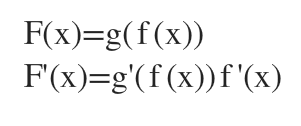# Suppose F(x) = g(f(x)) and f(2) = 3, f'(2) = -3, g(3) = 5, g'(3) = 4. Find F'(2). We are currently using the chain rule h(x) = g(f(x)) h'(x) = g'(f(x))f'(x) The answer should be -12 but I have no clue how to set a problem like this up.

Question
1 views

Suppose F(x) = g(f(x)) and f(2) = 3, f'(2) = -3, g(3) = 5, g'(3) = 4. Find F'(2).

We are currently using the chain rule h(x) = g(f(x)) h'(x) = g'(f(x))f'(x)

The answer should be -12 but I have no clue how to set a problem like this up.

check_circle

Step 1

We have to find F'(x) first using chain rule....

### Want to see the full answer?

See Solution

#### Want to see this answer and more?

Solutions are written by subject experts who are available 24/7. Questions are typically answered within 1 hour.*

See Solution
*Response times may vary by subject and question.
Tagged in